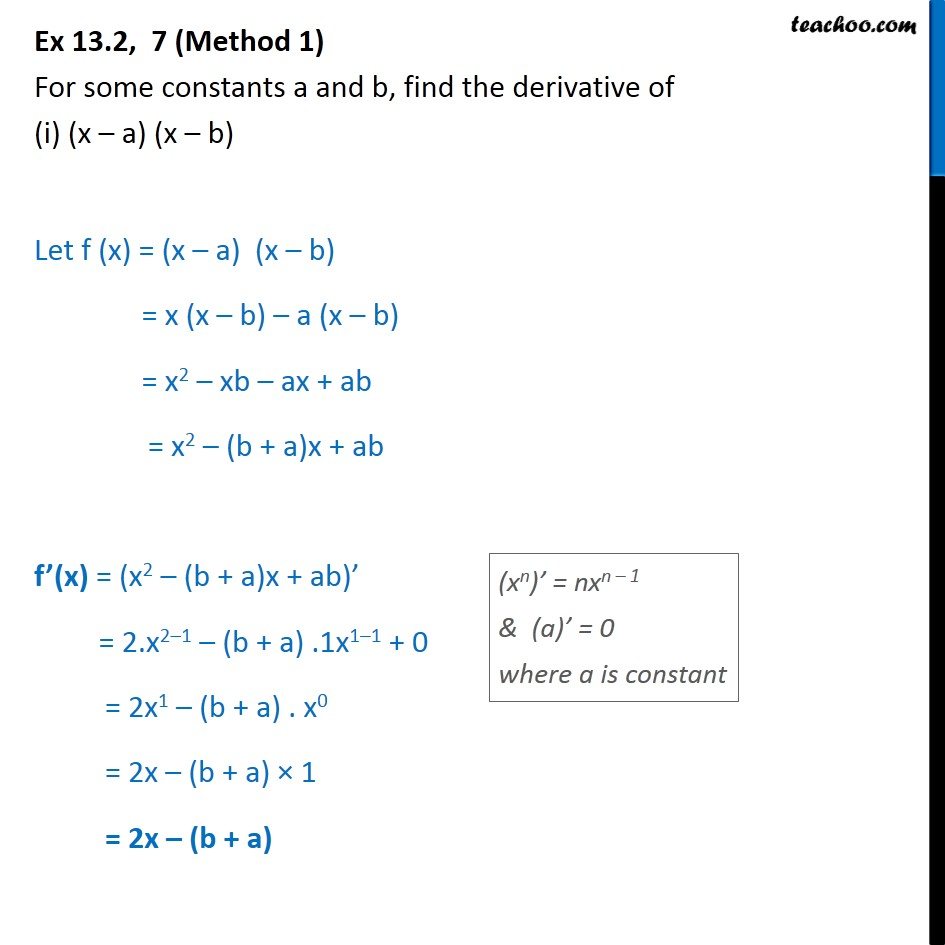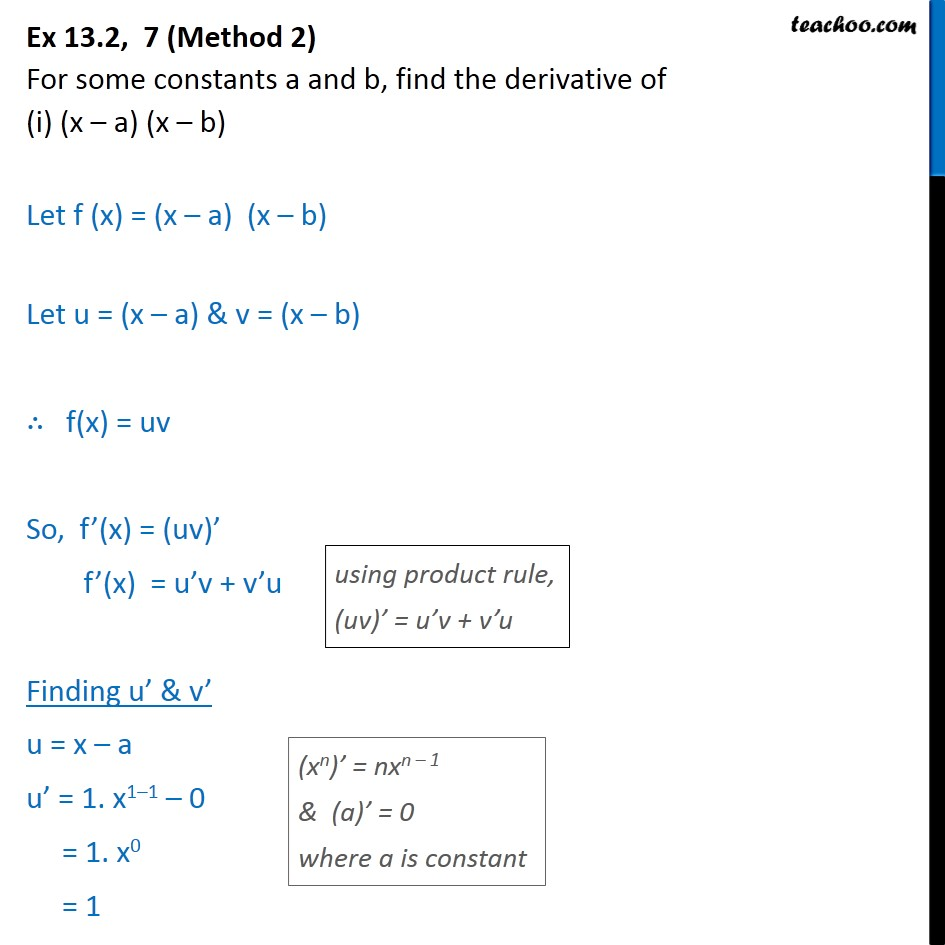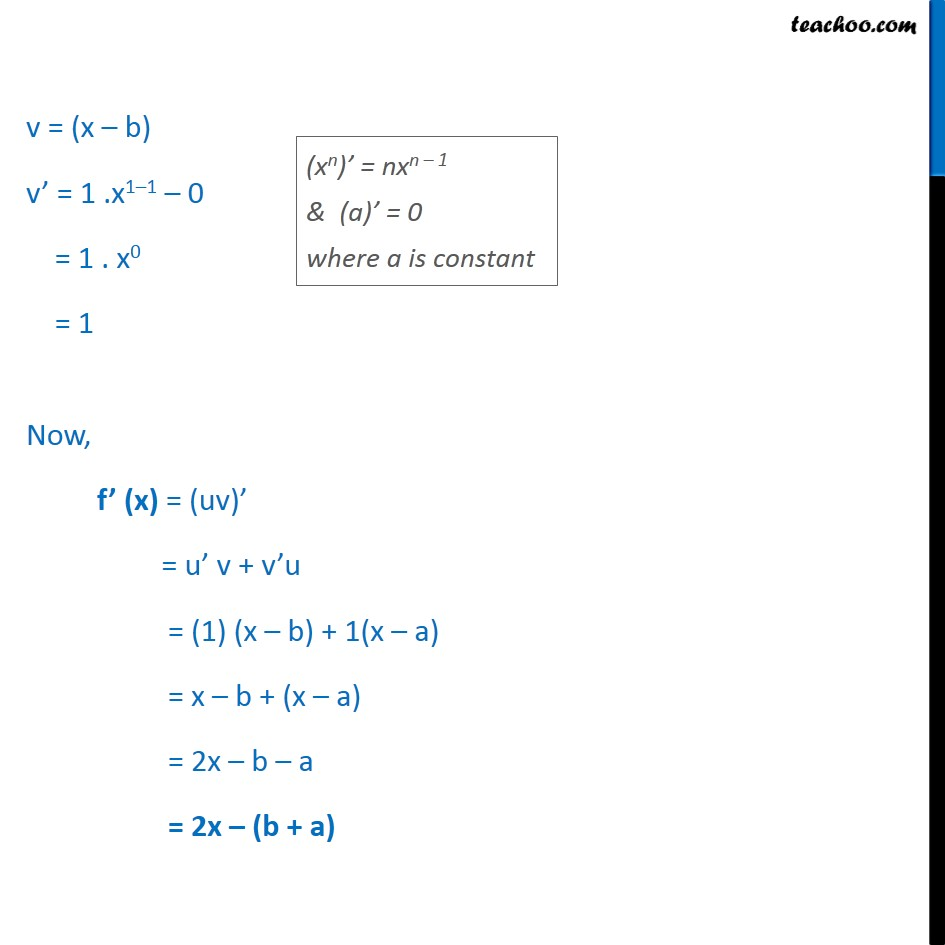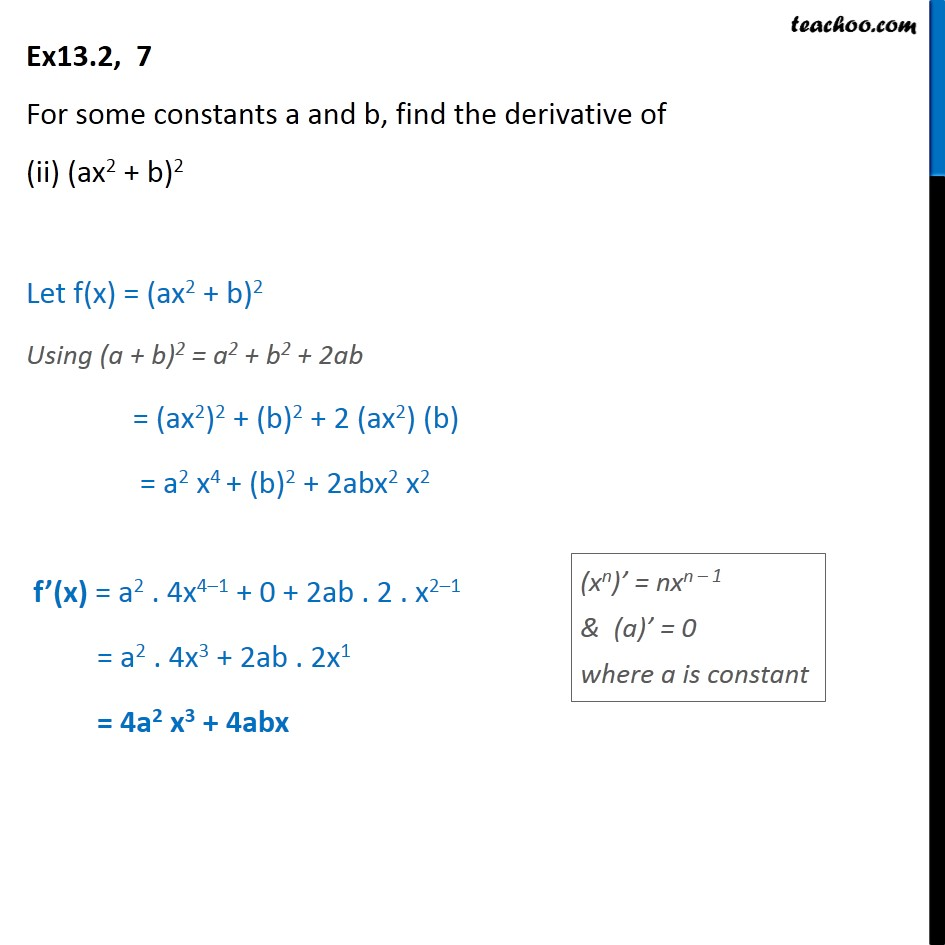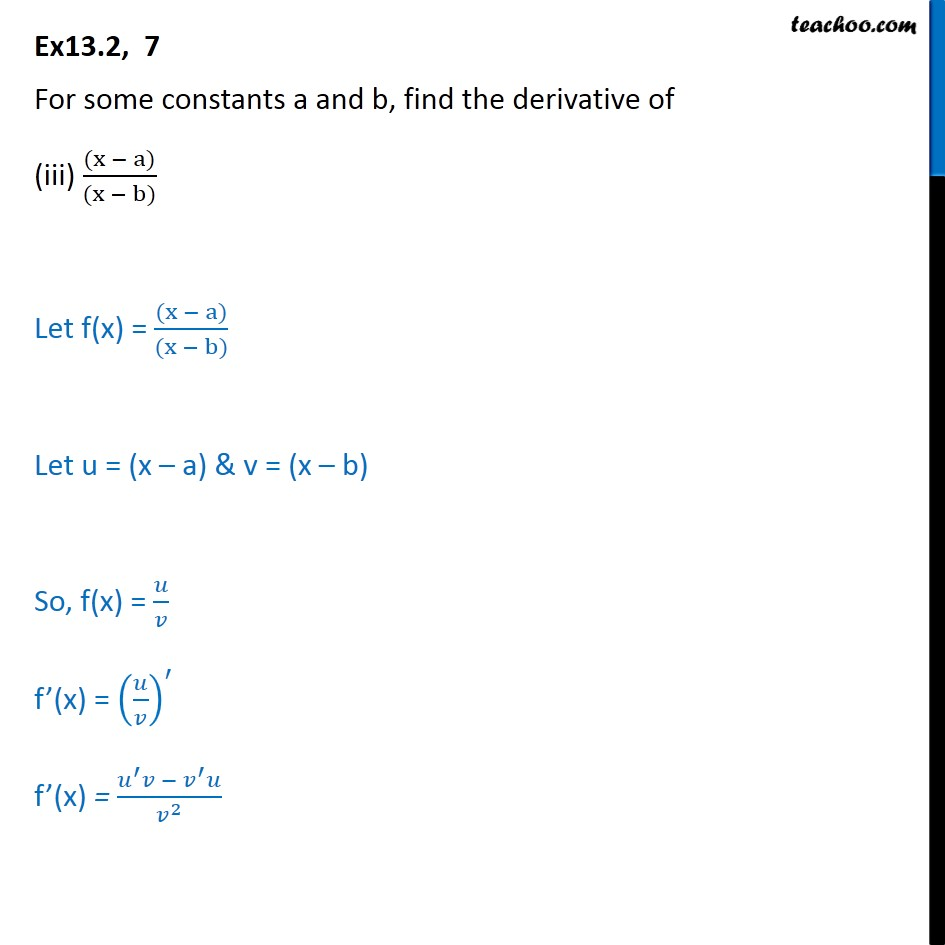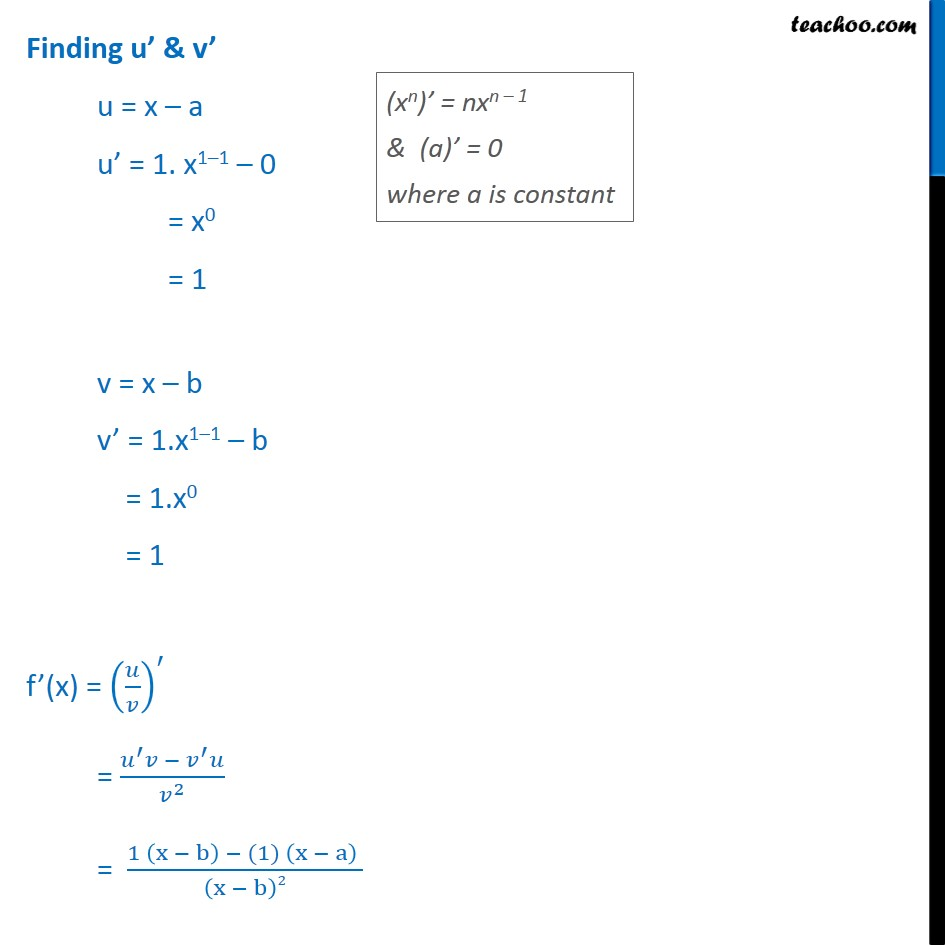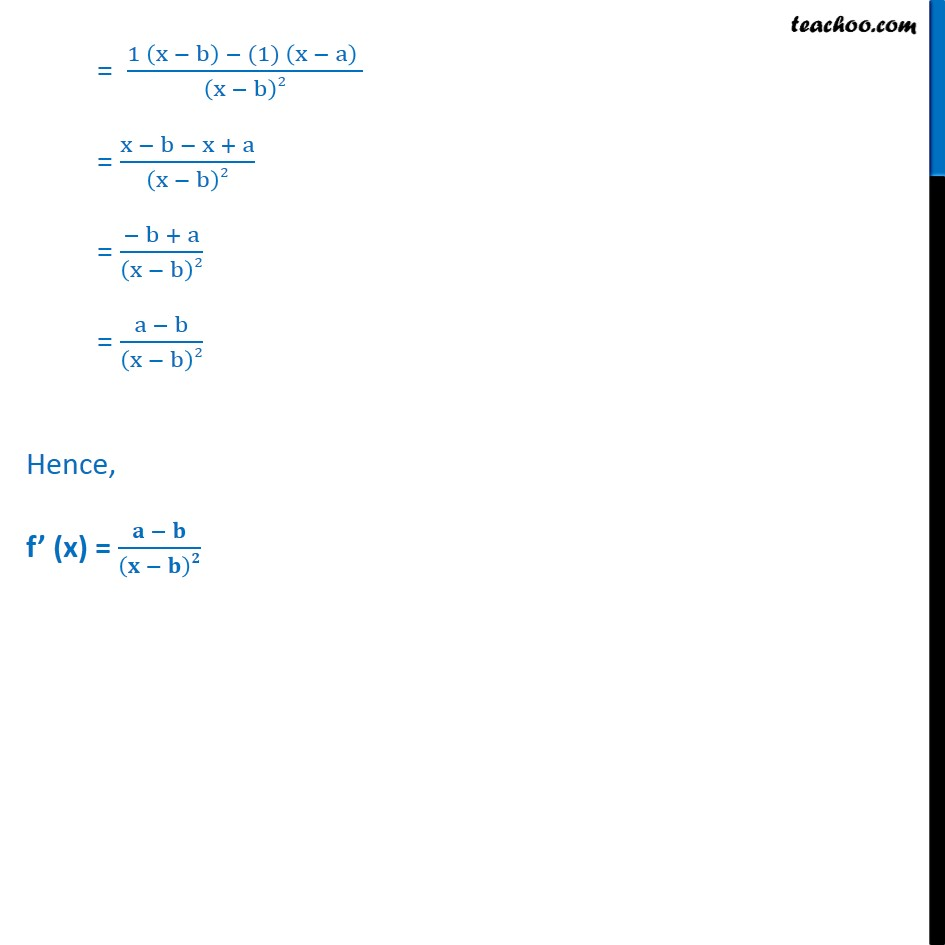1. Chapter 13 Class 11 Limits and Derivatives
2. Serial order wise
3. Ex 13.2

Transcript

Ex 13.2, 7 (Method 1) For some constants a and b, find the derivative of (i) (x – a) (x – b) Let f (x) = (x – a) (x – b) = x (x – b) – a (x – b) = x2 – xb – ax + ab = x2 – (b + a)x + ab f’(x) = (x2 – (b + a)x + ab)’ = 2.x2–1 – (b + a) .1x1–1 + 0 = 2x1 – (b + a) . x0 = 2x – (b + a) × 1 = 2x – (b + a) Ex 13.2, 7 (Method 2) For some constants a and b, find the derivative of (i) (x – a) (x – b) Let f (x) = (x – a) (x – b) Let u = (x – a) & v = (x – b) ∴ f(x) = uv So, f’(x) = (uv)’ f’(x) = u’v + v’u Finding u’ & v’ u = x – a u’ = 1. x1–1 – 0 = 1. x0 = 1 v = (x – b) v’ = 1 .x1–1 – 0 = 1 . x0 = 1 Now, f’ (x) = (uv)’ = u’ v + v’u = (1) (x – b) + 1(x – a) = x – b + (x – a) = 2x – b – a = 2x – (b + a) Ex13.2, 7 For some constants a and b, find the derivative of (ii) (ax2 + b)2 Let f(x) = (ax2 + b)2 Using (a + b)2 = a2 + b2 + 2ab = (ax2)2 + (b)2 + 2 (ax2) (b) = a2 x4 + (b)2 + 2abx2 x2 f’(x) = a2 . 4x4–1 + 0 + 2ab . 2 . x2–1 = a2 . 4x3 + 2ab . 2x1 = 4a2 x3 + 4abx Ex13.2, 7 For some constants a and b, find the derivative of (iii) (x − a)﷮(x − b)﷯ Let f(x) = (x − a)﷮(x − b)﷯ Let u = (x – a) & v = (x – b) So, f(x) = 𝑢﷮𝑣﷯ f’(x) = 𝑢﷮𝑣﷯﷯﷮′﷯ f’(x) = 𝑢﷮′﷯𝑣 − 𝑣﷮′﷯𝑢﷮ 𝑣﷮2﷯﷯ Finding u’ & v’ u = x – a u’ = 1. x1–1 – 0 = x0 = 1 v = x – b v’ = 1.x1–1 – b = 1.x0 = 1 f’(x) = 𝑢﷮𝑣﷯﷯﷮′﷯ = 𝑢﷮′﷯𝑣 − 𝑣﷮′﷯𝑢﷮ 𝑣﷮2﷯﷯ = 1 x − b﷯ − (1) x − a﷯ ﷮ x − b﷯2﷯ = 1 x − b﷯ − (1) x − a﷯ ﷮ x − b﷯2﷯ = x − b − x + a﷮ x − b﷯2﷯ = − b + a﷮ x − b﷯2﷯ = a − b﷮ x − b﷯2﷯ Hence, f’ (x) = 𝐚 − 𝐛﷮ 𝐱 − 𝐛﷯𝟐﷯

Ex 13.2

About the AuthorDavneet Singh
Davneet Singh is a graduate from Indian Institute of Technology, Kanpur. He has been teaching from the past 10 years. He provides courses for Maths and Science at Teachoo.